CompressibilityBy Wikipedia,
the free encyclopedia,

https://en.wikipedia.org/wiki/Compressibility

In thermodynamics and fluid mechanics, compressibility (also known as the coefficient of compressibility or isothermal compressibility) is a measure of the relative volume change of a fluid or solid as a response to a pressure (or mean stress) change.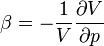where V is volume and p is pressure.

The minus sign makes the compressibility positive in the (usual) case that an increase in pressure induces a reduction in volume.

## Definition

The specification above is incomplete, because for any object or system the magnitude of the compressibility depends strongly on whether the process is adiabatic or isothermal. Accordingly, isothermal compressibility is defined:where the subscript T indicates that the partial differential is to be taken at constant temperature.

Isentropic compressibility is defined: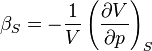where S is entropy. For a solid, the distinction between the two is usually negligible.

### Relation to speed of sound

The speed of sound is defined in classical mechanics as: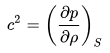where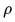is the density of the material. It follows, by replacing partial derivatives, that the isentropic compressibility can be expressed as: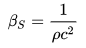### Relation to bulk modulus

The inverse of the compressibility is called the bulk modulus, often denoted K (sometimes B). That page also contains some examples for different materials.

The compressibility equation relates the isothermal compressibility (and indirectly the pressure) to the structure of the liquid.

## Thermodynamics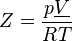where p is the pressure of the gas, T is its temperature, and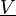is its molar volume. In the case of an ideal gas, the compressibility factor Z is equal to unity, and the familiar ideal gas law is recovered: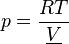Z can, in general, be either greater or less than unity for a real gas.

The deviation from ideal gas behavior tends to become particularly significant (or, equivalently, the compressibility factor strays far from unity) near the critical point, or in the case of high pressure or low temperature. In these cases, a generalized compressibility chart or an alternative equation of state better suited to the problem must be utilized to produce accurate results.

A related situation occurs in hypersonic aerodynamics, where dissociation causes an increase in the “notional” molar volume, because a mole of oxygen, as O2, becomes 2 moles of monatomic oxygen and N2 similarly dissociates to 2N. Since this occurs dynamically as air flows over the aerospace object, it is convenient to alter Z, defined for an initial 30 gram mole of air, rather than track the varying mean molecular weight, millisecond by millisecond. This pressure dependent transition occurs for atmospheric oxygen in the 2500 K to 4000 K temperature range, and in the 5000 K to 10,000 K range for nitrogen.

In transition regions, where this pressure dependent dissociation is incomplete, both beta (the volume/pressure differential ratio) and the differential, constant pressure heat capacity greatly increase.

For moderate pressures, above 10,000 K the gas further dissociates into free electrons and ions. Z for the resulting plasma can similarly be computed for a mole of initial air, producing values between 2 and 4 for partially or singly ionized gas. Each dissociation absorbs a great deal of energy in a reversible process and this greatly reduces the thermodynamic temperature of hypersonic gas decelerated near the aerospace object. Ions or free radicals transported to the object surface by diffusion may release this extra (non-thermal) energy if the surface catalyzes the slower recombination process.

The isothermal compressibility is related to the isentropic (or adiabatic) compressibility by the relation,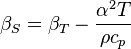via Maxwell’s relations. More simply stated,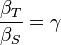where,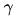is the heat capacity ratio. See here for a derivation.

Compressibility of ionic liquids and molten salts can be expressed as a sum of the contribution of the ionic lattice and of the holes.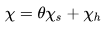## Earth science

Vertical, drained compressibilities
Material β (m²/N or Pa−1)
Plastic clay 2×10–6 – 2.6×10–7
Stiff clay 2.6×10–7 – 1.3×10–7
Medium-hard clay 1.3×10–7 – 6.9×10–8
Loose sand 1×10–7 – 5.2×10–8
Dense sand 2×10–8 – 1.3×10–8
Dense, sandy gravel 1×10–8 – 5.2×10–9
Ethyl alcohol 1.1×10–9
Carbon disulfide 9.3×10–10
Rock, fissured 6.9×10–10 – 3.3×10–10
Water at 25 °C (undrained) 4.6×10–10
Rock, sound <3.3×10–10
Glycerine 2.1×10–10
Mercury 3.7×10–11

The Earth sciences use compressibility to quantify the ability of a soil or rock to reduce in volume under applied pressure. This concept is important for specific storage, when estimating groundwater reserves in confined aquifers. Geologic materials are made up of two portions: solids and voids (or same as porosity). The void space can be full of liquid or gas. Geologic materials reduces in volume only when the void spaces are reduced, which expel the liquid or gas from the voids. This can happen over a period of time, resulting in settlement.

It is an important concept in geotechnical engineering in the design of certain structural foundations. For example, the construction of high-rise structures over underlying layers of highly compressible bay mud poses a considerable design constraint, and often leads to use of driven piles or other innovative techniques.

## Fluid dynamics

The degree of compressibility of a fluid has strong implications for its dynamics. Most notably, the propagation of sound is dependent on the compressibility of the medium.

### Aerodynamics

Compressibility is an important factor in aerodynamics. At low speeds, the compressibility of air is not significant in relation to aircraft design, but as the airflow nears and exceeds the speed of sound, a host of new aerodynamic effects become important in the design of aircraft. These effects, often several of them at a time, made it very difficult for World War II era aircraft to reach speeds much beyond 800 km/h (500 mph).

Many effects are often mentioned in conjunction with the term «compressibility», but regularly have little to do with the compressible nature of air. From a strictly aerodynamic point of view, the term should refer only to those side-effects arising as a result of the changes in airflow from an incompressible fluid (similar in effect to water) to a compressible fluid (acting as a gas) as the speed of sound is approached. There are two effects in particular, wave drag and critical mach.

## Negative compressibility

In general, the bulk compressibility (sum of the linear compressibilities on the three axes) is positive, i.e. an increase in pressure squeezes the material to a smaller volume. This condition is required for mechanical stability. However, under very specific conditions the compressibility can be negative.

Published in August 2018.